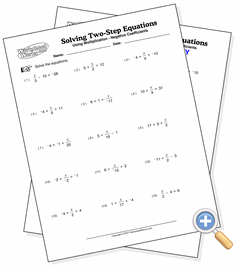# Using Multiplication

## Solving Two-Step EquationsSolve two-step equations using division

These two-step equations are solved by using addition and subtraction, then multiplication to eliminate a denominator. All problems resolve to integers. You can adjust the difficulty of the problems by increasing the values size, using negative coefficients and flipping the sides of the equations.